# Question about Biot-Savart Law (B-field)

## Homework Statement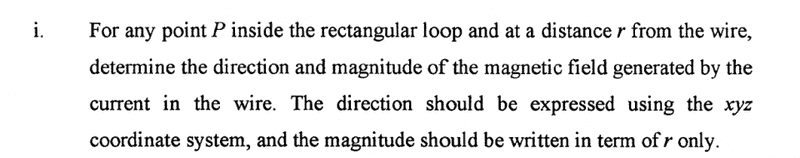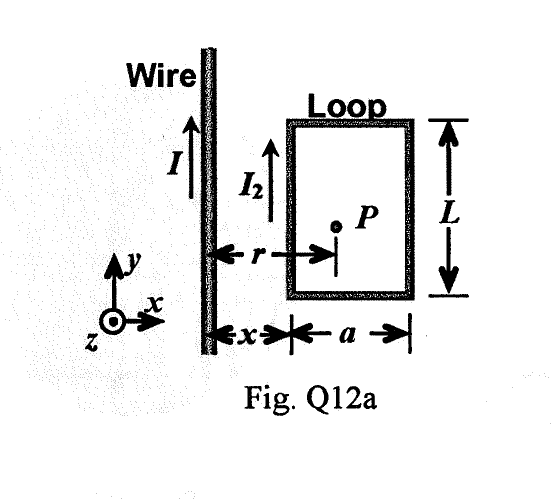I=10A,L=0.5m,a=0.3m,x=0.2m

## Homework Equations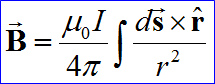## The Attempt at a Solution

by the Biot-Savart Law
ds cross r=-dxcosθ k(direction k)
dB= μI/4π∫-dxcosθ/r^2
B=μI/4π∫-dxcosθ/r^2 (from ?? to ??) in magnitude
question:
1) what is ?? to ??
2)how dx and r respect to dθ????

[/B]

#### Attachments

BvU
Homework Helper
Look up the magnetic field from a current carrying infinitely long straight wire, an application of the BS law.

Look up the magnetic field from a current carrying infinitely long straight wire, an application of the BS law.
where is the start and end point of integration? 0 to inf?
then change cosθ and r respect to x? also the direction B-field is -k?

BvU
Homework Helper
Are you aware of what you are doing ? What BS entails ? You write dx for an integration in the y direction, you write dB and on the next line B for the same expression on the right. You want to use the Biot Savart Law, which is fine, but somehat over the top: Ampere is already fine here!
where is the start and end point of integration? 0 to inf?
No, ##-\infty## to ##\infty##: everywhere where there is a contribution to the B field.

Are you aware of what you are doing ? What BS entails ? You write dx for an integration in the y direction, you write dB and on the next line B for the same expression on the right. You want to use the Biot Savart Law, which is fine, but somehat over the top: Ampere is already fine here!
No, ##-\infty## to ##\infty##: everywhere where there is a contribution to the B field.
OK thx

BvU
Homework Helper
Any idea what ##\theta ## is ?

Any idea what ##\theta ## is ?
Yes after check with my lecture notes and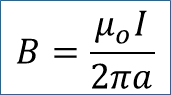for Magnetic Field for an Infinite Long, Straight Conductor

BvU
Homework Helper
Don't see no ##\theta##Can you derive ##B={\mu_0I\over 2\pi a}## from BS?

But I spy an ##a## and that's not the ##a## in 'your' problem statement!

Don't see no ##\theta##Can you derive ##B={\mu_0I\over 2\pi a}## from BS?

But I spy an ##a## and that's not the ##a## in 'your' problem statement!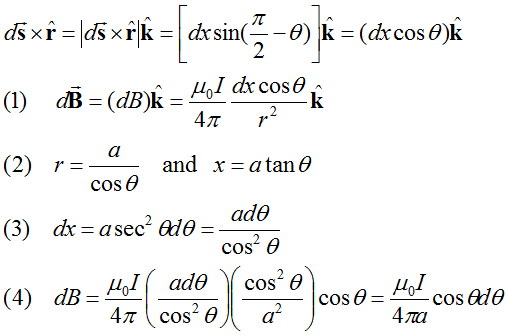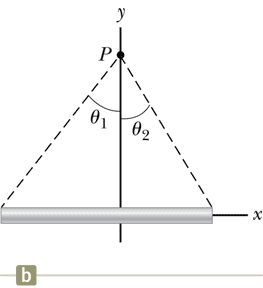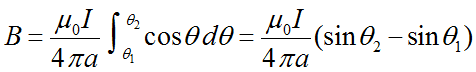θ1=-π/2,θ2=π/2,If the conductor is an infinitely long, straight wire
hencei think a is r when i used in this question

can i ask you another question?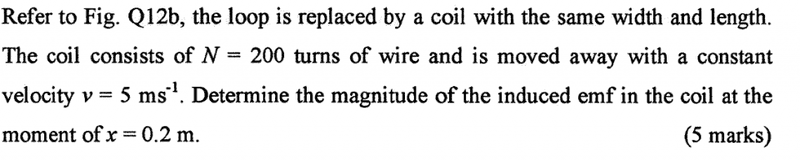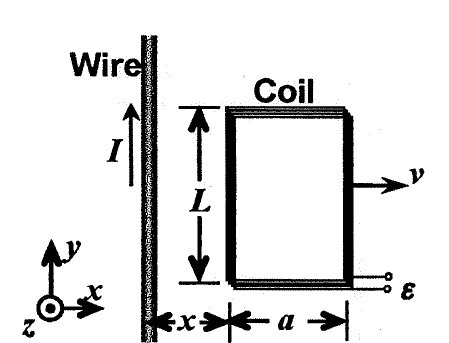using faraday law and lens law
e.m.f=-Nd(flux)/dt
=-200A(dB/dt)
=-200(aL)(dB/dt) how i link dB/dt with velocity dv/dt=5ms^-1???

BvU
Homework Helper
View attachment 99347View attachment 99349
View attachment 99348
θ1=-π/2,θ2=π/2,If the conductor is an infinitely long, straight wire
hence View attachment 99350
i think a is r when i used in this question
Reassuring you found it; especially the transition from ##dx## (your ##dy##) to ##d\theta## isn't all that trivial.
The end result is Ampere's law for the straight long wire: ##B \, 2\pi a = \mu_0 I##.
Good.

And yes, ##a=r##.

BvU
Homework Helper
can i ask you another question?
View attachment 99351 View attachment 99352
using faraday law and lens law
e.m.f=-Nd(flux)/dt
=-200A(dB/dt)
=-200(aL)(dB/dt) how i link dB/dt with velocity dv/dt=5ms^-1???
You use the result of part i.
And Flux is not ##AB## but ##\int \vec B(r)\cdot d\vec A\ \ ## !

You use the result of part i.
And Flux is not ##AB## but ##\int \vec B(r)\cdot d\vec A\ \ ## !
i feel confuse that what is the changing time in this question, since v=5ms^-1 with constant velocity,the question just asking the e.m.f at x=0.2m,but at the beginning of the figure ,it alreay in x=0.2m, so how can i cal?

i feel confuse that what is the changing time in this question, since v=5ms^-1 with constant velocity,the question just asking the e.m.f at x=0.2m,but at the beginning of the figure ,it alreay in x=0.2m, so how can i cal?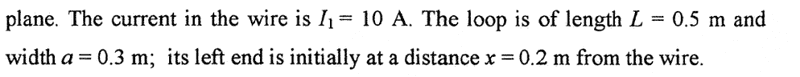BvU
Homework Helper
Do the integral for the flux. Should give you something that depends on x: ##\Phi(x)## . Then ##{d\over dt} \Phi = {d\over dx} \Phi \ {dx\over dt} = \Phi' v, \ \ ## to be evaluated at x = 0.2

Do the integral for the flux. Should give you something that depends on x: ##\Phi(x)## . Then ##{d\over dt} \Phi = {d\over dx} \Phi \ {dx\over dt} = \Phi' v, \ \ ## to be evaluated at x = 0.2
do you mean dr/dt in this case? since B=μI/2πr (dB/dr)(dr/dt)=(dr/dt)(-μI/2πr^2)=5(-μI/2π(0.2)^2) for x=0.2m

BvU
Homework Helper
No. For the flux, r is just an integration variable. ##v## is ##{dx\over dt} = 5 ## m/s.

No. For the flux, r is just an integration variable. ##v## is ##{dx\over dt} = 5 ## m/s.
i know the concept but i do not know how to solve
e.m.f=-Nd/dx(∫B dA) (5) then how can i solve it????

BvU
Homework Helper
Try to work out ##\int B \, dA ##

Note: e.m.f=-Nd/dt (∫B dA)

Try to work out ##\int B \, dA ##
B=
Try to work out ##\int B \, dA ##

Note: e.m.f=-Nd/dt (∫B dA)
∫B dA=∫μI/2πr^2 dA <----how A line with r dA=2πrdr?

B=

∫B dA=∫μI/2πr^2 dA <----how A line with r dA=2πrdr?
can you show me some step? i have no idea now...

BvU